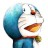# 杰拉斯的博客

## [ACM_HDU_2050]折线分割平面杰拉斯 | 时间：2012-03-28, Wed | 7,157 views

### 折线分割平面

Time Limit: 2000/1000 MS (Java/Others) Memory Limit: 65536/32768 K (Java/Others)
Total Submission(s): 8663 Accepted Submission(s): 6084

#### Description```2
1
2
```

```2
7
```

#### Source

HDU2050

@王xiao瓶 一同讨论了这道题，过程如下：

f(0) = 1
f(1) = 2
f(2) = 4
...
f(n) = f(n - 1) + n

f(n)
= f(n - 1) + n
= f(n - 2) + n + (n - 1)
= ...
= f(0) + n + (n - 1) + ... + 1
= 1 + (1 + n) * n / 2

f(0) = 1
f(1) = 4
f(2) = 11
...
f(n) = f(n - 1) + 2 * n - 1 + 2 * n = f(n - 1) + 4 * n - 1

f(n)
= f(n - 1) + (4 * n - 1)
= f(n - 2) + 4 * (n - 1) - 1 + (4 * n - 1)
= ...
= f(0) + (4 * 1 - 1) + (4 * 2 - 1) + ... + (4 * n - 1)
= 1 + 4 * (1 + 2 + .. + n) - n
= 1 + 4 * (1 + n) * n / 2 - n
= 1 + 2 * n * n + n

f(n)
= 1 + (1 + (2 * n)) * (2 * n) / 2
= 1 + 2 * n * n + n

f(n)
= f(n - 1) + 4 * n - 1 - 2
= f(n - 1) + 4 * n - 3

f(n)
= 1 + 2 * n * n + n - 2 * n
= 1 + 2 * n * n - n

```int main()
{
int i, n, a;
a = 2;
for(i = 2; i < 10001; ++i){
a[i] = a[i - 1] + 4 * i - 3;
}
cin >> n;
while(n--){
cin >> i;
cout << a[i] << endl;
}
return 0;
}
```

```int main()
{
int n, i;
cin >> n;
while(n--){
cin >> i;
cout << 2 * i * i - i + 1 << endl;
}
return 0;
}
```

#### 仅有一条评论 »

1.相框墙图片

博客知识很好哈，请问可以交换链接吗？